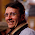## Friday, January 16, 2009

### Computing efficiently the Erdős numbers

From wikipedia: "In order to be assigned an Erdős number, an author must co-write a mathematical paper with an author with a finite Erdős number. Paul Erdős is the one person having an Erdős number of zero. If the lowest Erdős number of a coauthor is k, then the author's Erdős number is k + 1."
• You are given a collection of K papers, can you write an efficient algorithm for computing the Erdős number associated to each author?

#### 1 comment:

1.I just discovered I have an erdos number == 4